Next: Creating a Coarse-grained Model Up: INTRODUCTION Previous: INTRODUCTION   Contents

# The Force Field

A general force field can be written as a series of terms representing the interactions between increasingly large sets of atoms [34,35]: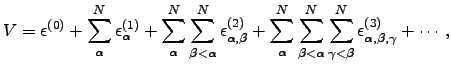(3.1)

where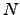is the total number of atoms, and the two-body term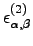, for instance, describes the interaction of two atoms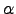and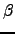.

Three-body and higher order terms in Equation 1.1 are often neglected, such as, for example, in the Lennard-Jones (LJ) pair potential [36,9], which takes the form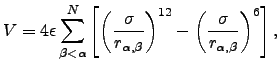(3.2)

where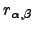is the distance between atomsand,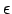is the depth of the potential energy well, and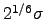is the pair equilibrium separation. This is an approximate potential as its form is a trade-off between the accurate reproduction of the interaction between closed-shell atoms and mathematical and computational simplicity. In this thesis we will use it to describe atomic clusters of various sizes.Next: Creating a Coarse-grained Model Up: INTRODUCTION Previous: INTRODUCTION   Contents
Semen A Trygubenko 2006-04-10